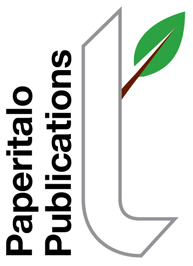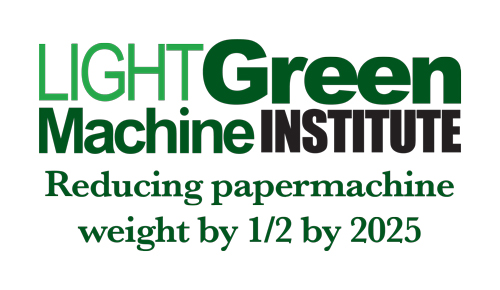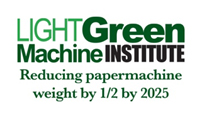The Light Green Machine Institute
 Quick LinksOctober 2019: The Optimization Problem--Clues to Solution, Part 3By Jim Thompson   Last month we moved from our hypothetical 2-D encapsulation problem ( August Column) to a hypothetical 3-D encapsulation problem ( September Column). Just so we don't forget the ultimate objective as we chase these hypothetical enclosures, is this:  What is the optimum paper machine building size (L x W x H) considering the static given of trim width and the dynamic of paper machine speed. Stated another way, when designing a new paper machine facility, what is the minimum possible expenditure on the building enclosing the paper machine for a given set of operating conditions?  You might note that I am doing this all with algebra, for two reasons (1) more readers likely understand algebra than calculus and (2) complex problems can often be developed in a clearer way using algebra. Let's move on. Thinking in practical terms for a minute, we know a paper machine's predominant dimension, even to the novice, is its length. Hence, our theoretical sphere from last month is not going to translate well to the practical.  So with a nod to the practical, we are going to have to come up with another solid shape, even in the theoretical, if we hope to ever have a solid practical solution. I've given this some thought, and two shapes come to mind.  The first is the old artist's trick of putting a model ship in a bottle.  A ship is long, tall, and wide.  A bottle works well to encapsulate it.   Another common shape here in North America, if you travel to rural areas, is the LPG (Liquid Propane Gas) tank found on farms and other homes remote from natural gas distribution systems.  This tank is cylindrical with spherical ends, designed to hold relatively high pressure contents. The LPG tank will make a good model for us (with or without the spherical ends). If we look at this in cross section, we seem to be back to our August Column, for in cross section a cylinder is a circle.  The area of the cross section is pi r^2 and the circumference is pi d where r is the radius and d is 2 x pi, or the diameter. Now if we let, nominally: W = trim width W = tending aisle 0.5 x W = drive aisle (I use non-dimensional "units" so that the reader may use metric or english, their preference) We find out that D = W + W + .5W = 2.5W and R = 2.5W/2 = 1.25W Hence, the circumference = pi x d = 7.85W (units) and the area of the cross section = pi x (1.25W)^2 = 4.91W^2 (units^2). We come to our first practical application.  Given the nominal trim width of your existing paper machine hall, you can calculate the encapsulated perimeter (height of two walls + width of roof + width of basement floor) and compare this to the ideal hypothetical for your machine.  Additionally, you can calculate the cross section of your existing machine hall and compare that to the hypothetical cross section we calculated above. Now, how long is our cylinder?  It depends on whether it is going to have flat or spherical ends (think back to the gas tank).  For the moment, we'll assume it is going to have flat ends. In order to define its length, we need to establish a few more dimensions.  These all run in the machine direction.  We will define them as follows: WE = the length of the Wet End of the machine from the wall to the slice lip of the Headbox. F = Fourdrinier length P = Press Section length PD = Pre Dryer Section Length S = Size Press Length PS = Post Size Press Dryer Section Length DE = the length of the Dry End from the last dryer to the wall. In the case of PD and PS, I am assuming cast iron dryers and their corresponding heat transfer coefficient as the standard.  We'll introduce a method to account for other materials later. With simple arithmetic, then, the length of the machine hall is WE+F+P+PD+S+PS+DE. And to clean up our nomenclature housekeeping here, we will define T = Total Length =  WE+F+P+PD+S+PS+DE (units). Now, the volume of our theoretical machine hall is T x 4.91 W^2 or, 4.91TW^2 (units^3). The area of the floor is T x D = 2.5TW (units^2). And the cylinder surface area is  T x 7.85W = 7.85TW (units^2) plus the two ends (2 x 4.91W^2) = 7.85TW + 9.82 W^2  (units^2). Check my math and forward any corrections, please! Check a couple of machine halls for which you have the dimensions and see how they come out compared to this theoretical calculation.  Please pass them along (keep the actual machines confidential). Next, month, we'll make it move--introducing the dynamics of paper machine speed! To be continued...
 As always, your comments will be appreciated. Think light! Jim Thompson Founder _____   Send us your comments by emailing Jim Thompson
 Support the Light Green Machine Institute every time you shop at Amazon. We have been accepted by Amazon for their smile.amazon program.  Every time you shop at Amazon on the smile.amazon site, you can support the LGMI.  Just follow this link to set this up.
 The Light Green Machine Institute is a 501C3 Delaware Registered Corporation. If you are interested in making tax deductible donations to our initiatives, please email jthompson@taii.com for information.